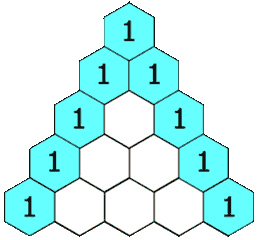# LeetCode 118. Pascal’s Triangle

## Description

https://leetcode.com/problems/pascals-triangle/

Given a non-negative integer numRows, generate the first numRows of Pascal’s triangle.In Pascal’s triangle, each number is the sum of the two numbers directly above it.

Example:

```Input: 5
Output:
[
,
[1,1],
[1,2,1],
[1,3,3,1],
[1,4,6,4,1]
]```

## Explanation

use a dynamic programming approach to constructing Pascal’s triangle each row based on the previous row.

## Python Solution

``````class Solution:
def generate(self, numRows: int) -> List[List[int]]:
result = []

if numRows < 1:
return result

for i in range(0, numRows):
row = []

if i == 0:
row.append(1)
else:
row.insert(0, 1)
row.insert(i, 1)

for j in range(1, i):
left_above = result[i - 1][j  - 1]
right_above = result[i - 1][j]
row.insert(j, left_above + right_above)

result.append(row)

return result``````
• Time complexity: O(numRows^2).
• Space complexity: O(numRows^2).